# Why Do We Plot Predictions on the x-axis?

When studying regression models, One of the first diagnostic plots most students learn is to plot residuals versus the model’s predictions (that is, with the predictions on the x-axis). Here’s a basic example.

``````# build an "ideal" linear process.
set.seed(34524)
N = 100
x1 = runif(N)
x2 = runif(N)
noise = 0.25*rnorm(N)
y = x1 + x2 + noise
df = data.frame(x1=x1, x2=x2, y=y)

# Fit a linear regression model
model = lm(y~x1+x2, data=df)
summary(model)``````
``````##
## Call:
## lm(formula = y ~ x1 + x2, data = df)
##
## Residuals:
##      Min       1Q   Median       3Q      Max
## -0.73508 -0.16632  0.02228  0.19501  0.55190
##
## Coefficients:
##             Estimate Std. Error t value Pr(>|t|)
## (Intercept)  0.16706    0.07111   2.349   0.0208 *
## x1           0.90047    0.09435   9.544 1.30e-15 ***
## x2           0.81444    0.09288   8.769 6.07e-14 ***
## ---
## Signif. codes:  0 '***' 0.001 '**' 0.01 '*' 0.05 '.' 0.1 ' ' 1
##
## Residual standard error: 0.2662 on 97 degrees of freedom
## Multiple R-squared:  0.6248, Adjusted R-squared:  0.6171
## F-statistic: 80.78 on 2 and 97 DF,  p-value: < 2.2e-16``````
``````# plot it
library(ggplot2)

df\$pred = predict(model, newdata=df)
df\$residual = with(df, y-pred)

# standard residual plot
ggplot(df, aes(x=pred, y=residual)) +
geom_point(alpha=0.5) + geom_hline(yintercept=0, color="red") +
geom_smooth(se=FALSE) +
ggtitle("Standard residual plot",
subtitle = "linear model and process")``````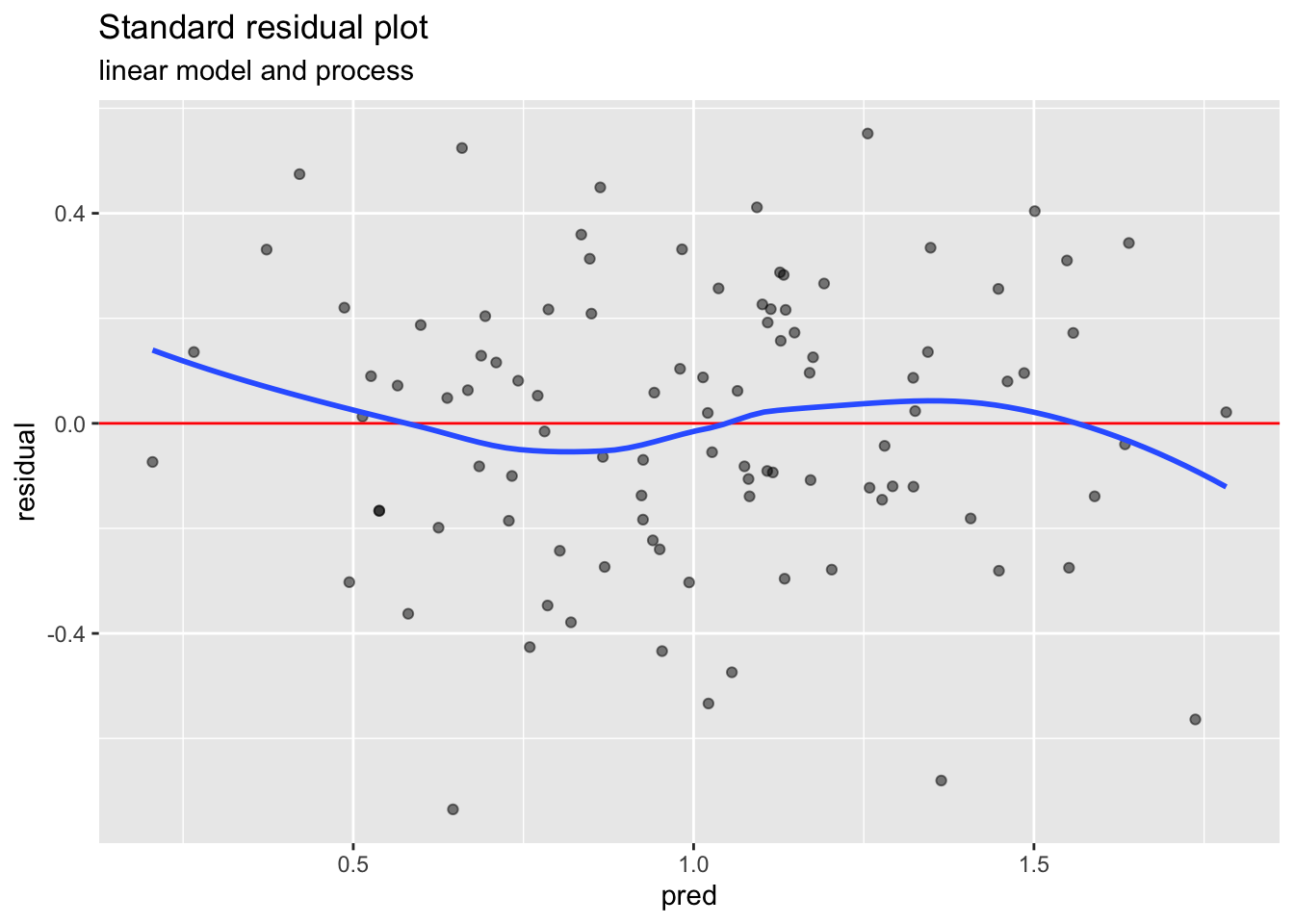In the above plot, we’re plotting the residuals as a function of model prediction, and comparing them to the line `y = 0`, using a smoothing curve through the residuals. The idea is that for a well-fit model, the smoothing curve should approximately lie on the line `y = 0`. This is true not only for linear models, but for any model that captures most of the explainable variance, and for which the unexplainable variance (the noise) is IID and zero mean.

If the residuals aren’t zero mean independently of the model’s predictions, then either you are missing some explanatory variables, or your model does not have the correct structure, or an appropriate inductive bias. A simple example of the second case is trying to fit a linear model to a process where the outcome is quadratically (or otherwise non-linearly) related to the outcome. To see this, let’s make an example quadratic system while deliberately failing to supply that structure to the model.

``````# a simple quadratic example
x3 = runif(N)
qf = data.frame(x1=x1, x2=x2, x3=x3)
qf\$y = x1 + x2 + 2*x3^2 + 0.25*noise

# Fit a linear regression model
model2 = lm(y~x1+x2+x3, data=qf)
# summary(model2)

qf\$pred = predict(model2, newdata=qf)
qf\$residual = with(qf, y-pred)

ggplot(qf, aes(x=pred, y=residual)) +
geom_point(alpha=0.5) + geom_hline(yintercept=0, color="red") +
geom_smooth(se=FALSE) +
ggtitle("Standard residual plot",
subtitle = "linear model, quadratic process")``````In this case, the smoothing line on the residuals doesn’t approximate the line `y = 0`; when the model predicts a value in the range 1 to about 2.3, it tends to be overpredicting; otherwise, it tends to underpredict. This is an instance of a pathology called “structure in the residuals.”

### The Peril of Outcomes on the x-axis

What happens if you erroneously plot the residuals versus the true outcome, instead of the predictions? Let’s try this with the model for the linear process (which we know is a well-fit model):

``````# the wrong residual graph
ggplot(df, aes(x=y, y=residual)) +
geom_point(alpha=0.5) + geom_hline(yintercept=0, color="red") +
geom_smooth(se=FALSE) +
ggtitle("Incorrect residual plot",
subtitle = "linear model and process")``````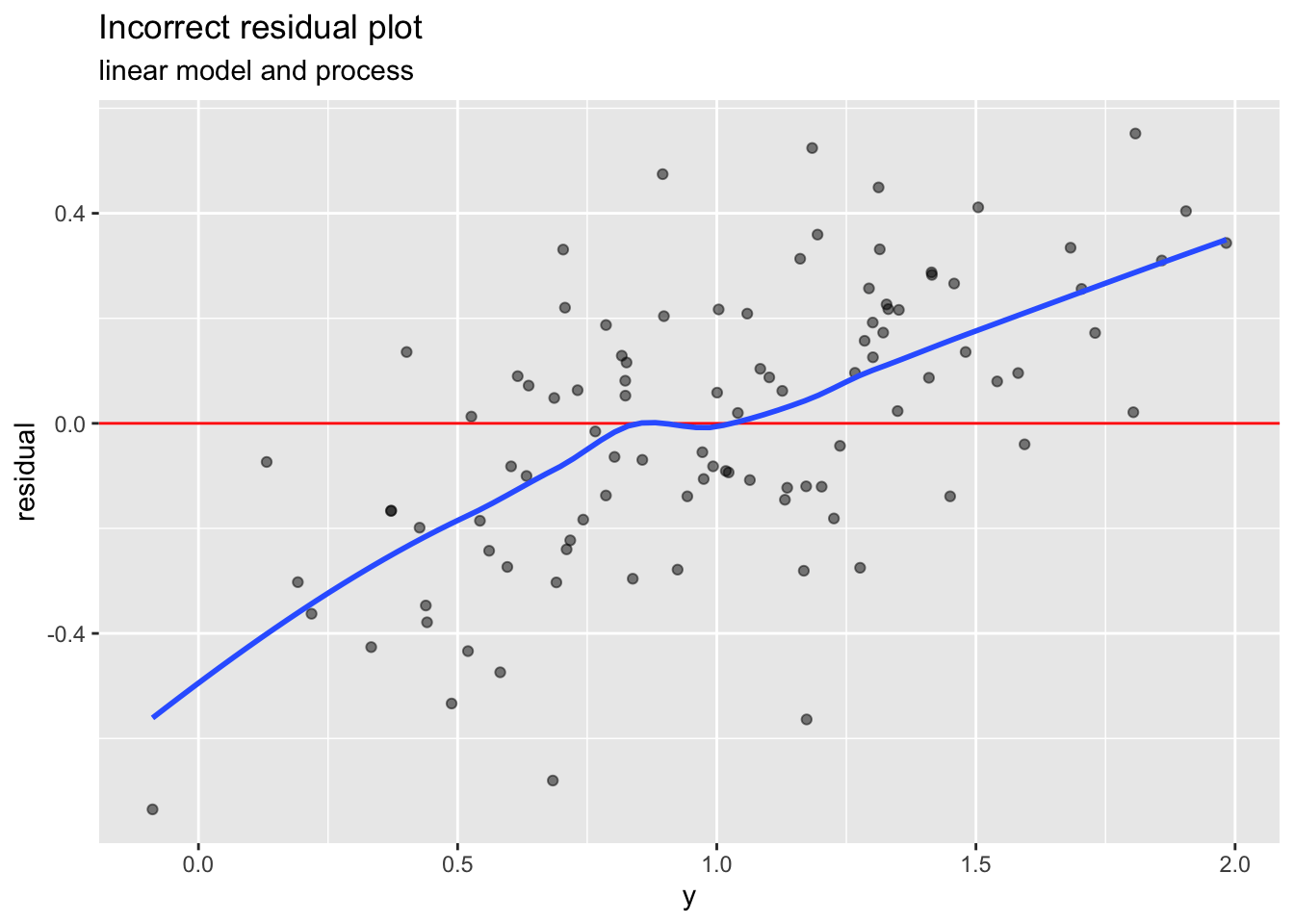If you make this plot when you meant to make the other, you will give yourself a nasty shock. Plotting residuals versus the outcome will always look more or less like the above graph. You might think that for a good model, the outcome and the prediction are close to each other, so the residual graphs should look about the same no matter which quantity you plot on the x-axis, right? Why do they look so different?

### Reversion to mediocrity (or the mean)

One reason that the proper residual graph (for a well fit model) should smooth out to the line `y=0` is known as reversion to mediocrity, or regression to the mean.

Imagine that you have an ideal process that always produces a single value y. You don’t actually observe this “true value”; instead, what you observe is y plus (IID, zero mean) noise. You can build a “model” for this process that predicts the mean of the observations, in this case the value 0.1033149. Then you can calculate the residuals of your “model” in the usual way.

When you plot the residuals as a function of the prediction, all the datums fall at the same horizontal coordinate of the graph, centered around zero, and approximately equally distributed between positive and negative. The “smoothing line” through this graph is simply the point (0.1033149, 0) – that is, the graph is centered at zero.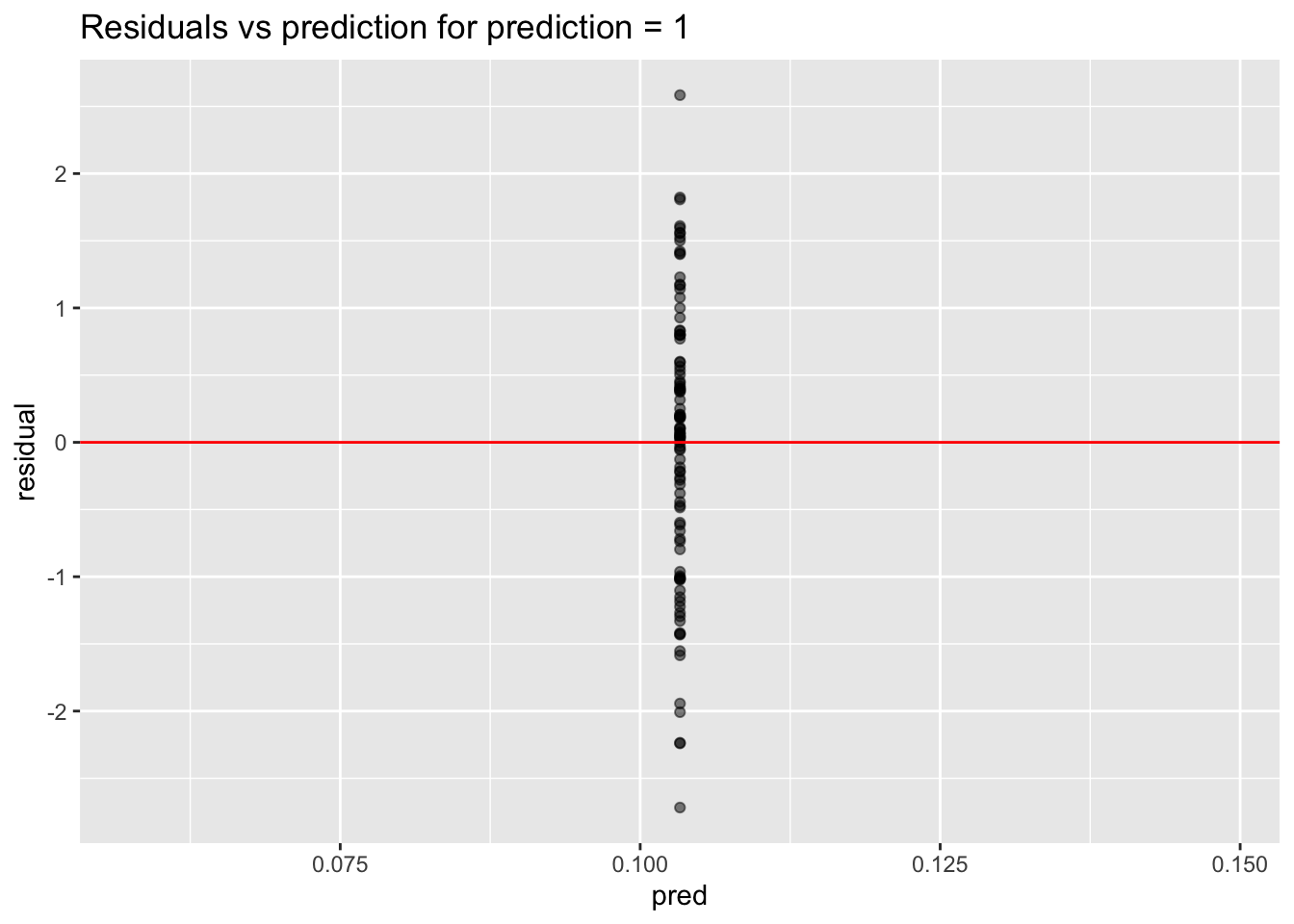On the other hand, if you plot the residuals as a function of the observed outcome, all the observations will be sorted so that the observations with positive noise are to the right of the observations with negative noise, and the smoothing line through the graph no longer looks like the line `y = 0`.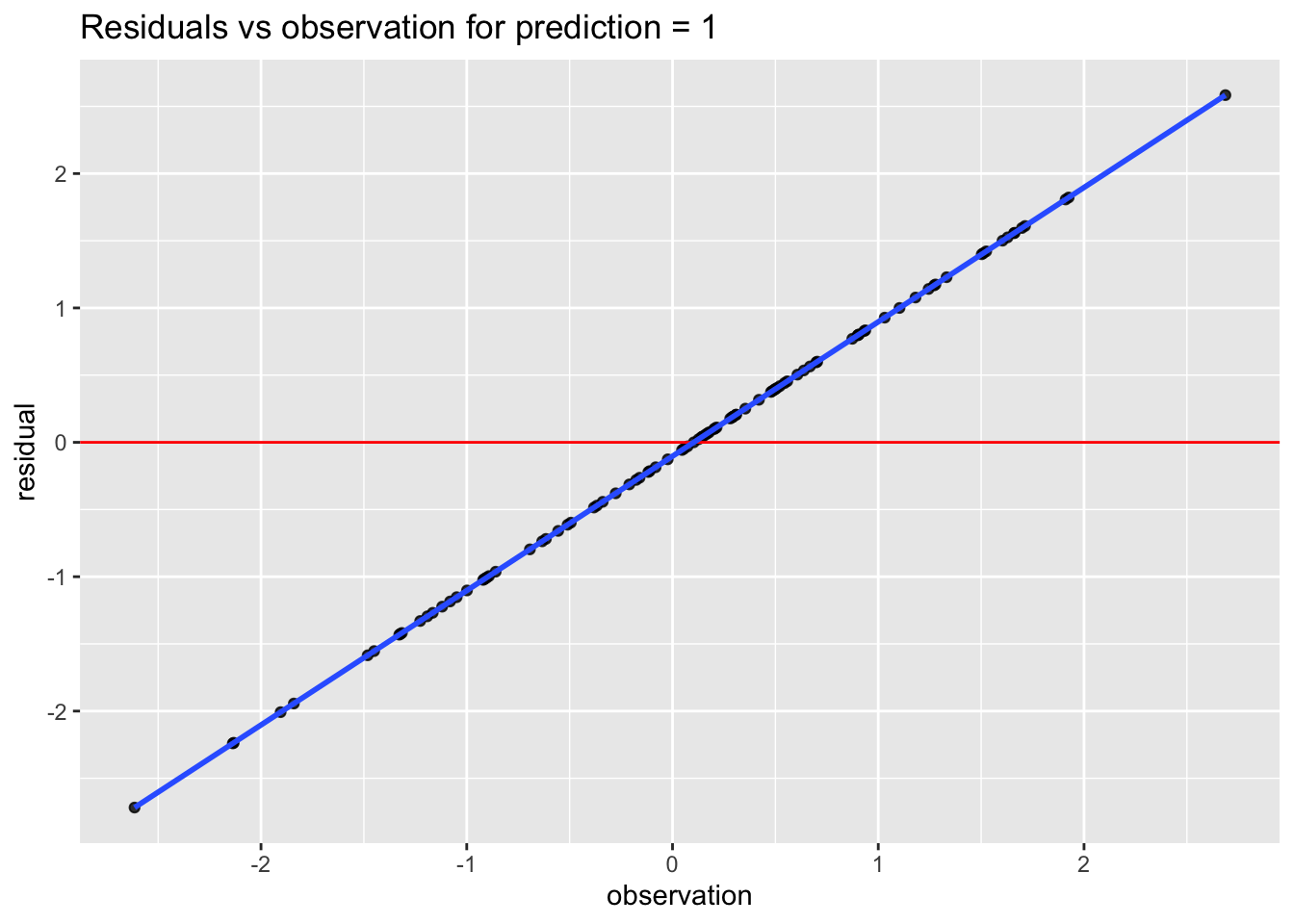For a process that varies as a function of the input, you can think of the prediction corresponding to an input `X` as the mean of all the observations corresponding to `X`, and the idea is the same.

Incidentally, this regression to the mean is also why model predictions tend to have less range than the original training data.

### Plotting observations versus predictions

Sometimes instead of plotting residuals versus the predictions, I plot observations versus predictions. In this case, you want to check that the predictions lie approximately on the line `y = x`. This isn’t a standard diagnostic plot, but it does give a better sense of the magnitude of the errors relative to the magnitudes of the outcomes. Again, the important thing to remember is that the predictions go on the x-axis.

Here’s the correct plot:

``````# standard prediction plot
ggplot(df, aes(x=pred, y=y)) +
geom_point(alpha=0.5) + geom_abline(color="red") +
geom_smooth(se=FALSE) +
ggtitle("Standard prediction plot")``````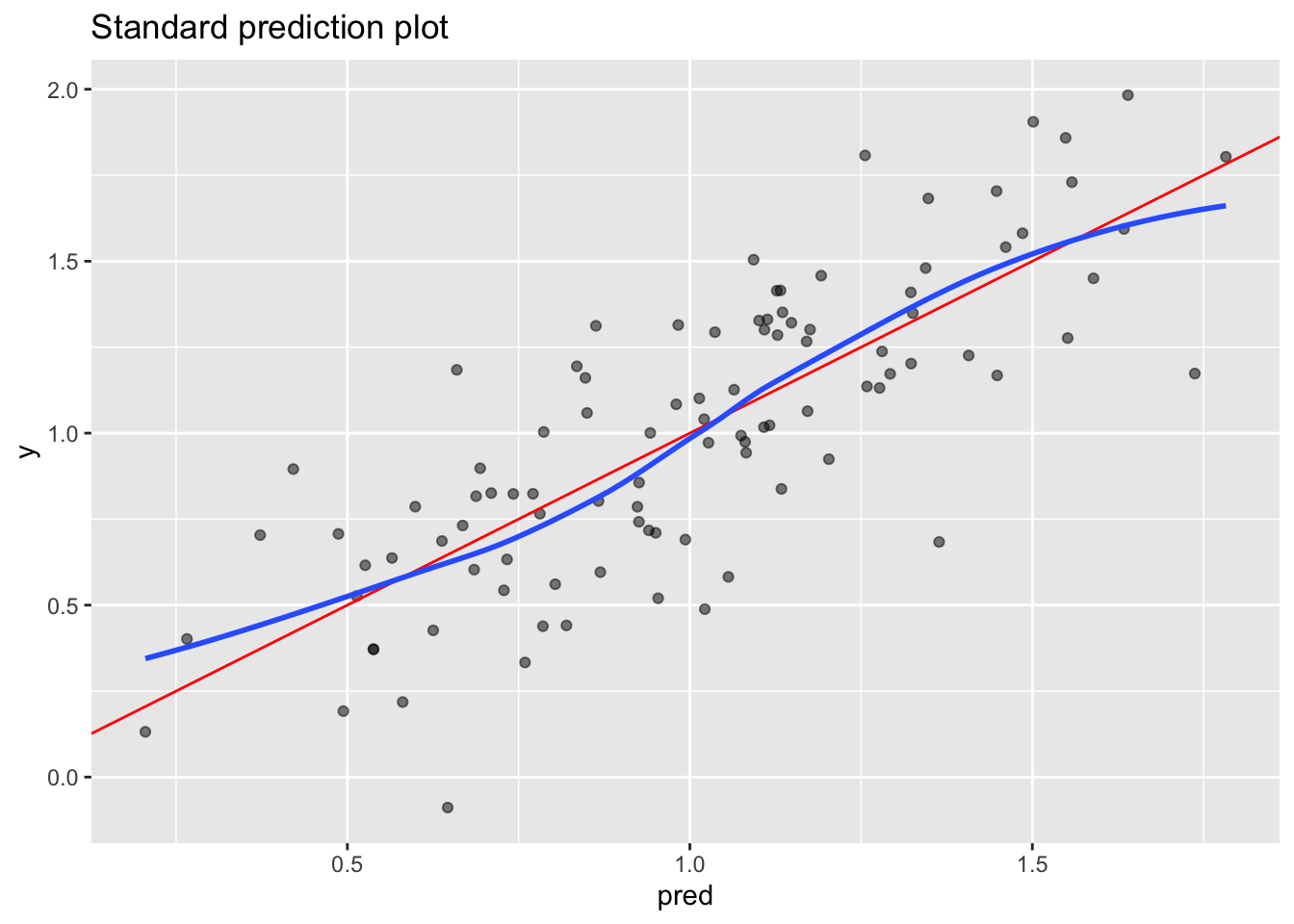And here’s the wrong plot:

``````# the "wrong" way
ggplot(df, aes(x=y, y=pred)) +
geom_point(alpha=0.5) + geom_abline(color="red") +
geom_smooth(se=FALSE) +
ggtitle("Incorrect prediction plot")``````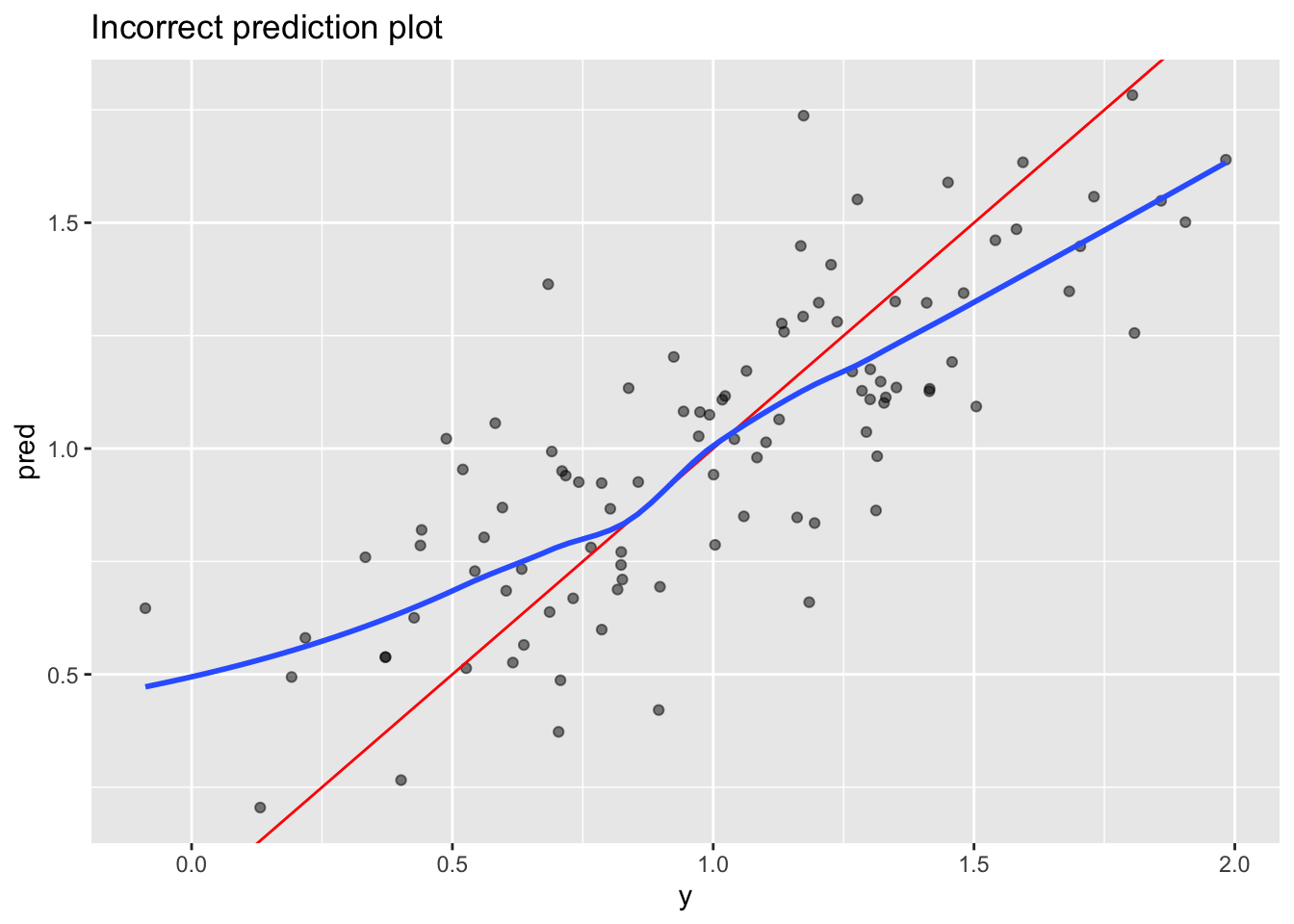Notice how the wrong plot again seems to show pathological structure where none exists.

### Conclusion

The above examples show why you should always take care to plot your model diagnostics as functions of the predictions and not of the observations. Most students have heard this already, but we feel that demonstrating why will be more memorable that simply saying “make it so.”

Tagged as:### Nina Zumel

Data scientist with Win Vector LLC. I also dance, read ghost stories and folklore, and sometimes blog about it all.